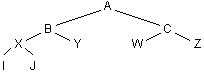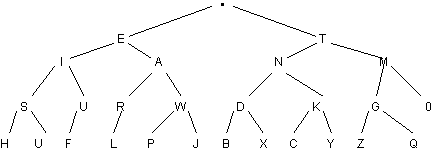Exercises
Trees

Use the figure below to answer the following questions.1. What is the root?
2. What is the height of the tree?
3. List the descendants of B.
4. List the siblings of W.
5. List the children of C.
6. List the ancestors of Z.
7. What is the parent of J?
8. Which nodes are leaves?
9. What is the level of W?
10. What is the depth of Y?
11. What is the maximum number of nodes in a binary tree of
• height 3?
• height 10?
• height h?
12. What is the maximum number of leaves in a binary tree of
• height 3?
• height 10?
• height h?
13. Draw the tree indicated by this array:

 L R 1 4 0 2 0 5 3 2 1 4 0 0 5 6 0 6 0 0

14. What does this tree represent in the "real world?"Continue to:  Unit 8 / Prev / Next